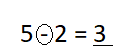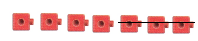Refer to our Texas Go Math Grade 1 Answer Key Pdf to score good marks in the exams. Test yourself by practicing the problems from Texas Go Math Grade 1 Module 5 Assessment Answer Key.

Vocabulary

Circle the minus sign. (p.166)
Underline the difference. (p.166)

Question 1.
5 – 2 = 3Explanation:
Circled the minus sign. Underlined the difference.

Concepts and Skills

Circle the part you are taking from, or separating from, the group. Then cross it out. Write how many there are now. TEKS 1.3.B

Question 2.There are 7 birds.
3 birds fly away.
How many birds are there now?
___ birds nowUseto show taking from, or separating from. Draw the. Circle the part you take away from, or separate from, the group. Then cross it out. Write the difference. TEKS 1.3.B

Question 3.
6 cats 3 cats run away.
6 – 3 = __Explanation:
Difference of 6 and 3 is 3

Question 4.
5 dogs 4 dogs run away.
5 – 4 = ___Explanation:
Difference of 5 and 4 is 1
Texas Test Prep

Question 5.
Which number sentence solves the problem? There are 6 toy cars. 4 toy cars are big. The rest are small.
How many toy cars are small? TEKS 1.3.B, 1.5.D
(A) 6 – 4 = 2
(B) 3 + 3 = 6
(C) 6 + 4 = 10
Explanation:
To find small cars, we need to minus the big cars from total cars

Question 6.
How many fewer bats are there? TEKS 1.3.B(A) 10 fewer
(B) 3 fewer
(C) 7 fewer
Explanation:
To find fewer bats, we need to minus the total bats from total balls
10 – 3 = 7

Question 7.
What number completes the subtraction sentence? TEKS 1.3.B
4 – ___ = 4
(A) 0
(B) 1
(C) 4